×#### Thank you for registering.

One of our academic counsellors will contact you within 1 working day.

Click to Chat

1800-1023-196

+91-120-4616500

CART 0

• 0

MY CART (5)

Use Coupon: CART20 and get 20% off on all online Study Material

ITEM
DETAILS
MRP
DISCOUNT
FINAL PRICE
Total Price: Rs.

There are no items in this cart.
Continue Shopping• Complete JEE Main/Advanced Course and Test Series
• OFFERED PRICE: Rs. 15,900
• View Details

```Solved Examples on Circular & Rotational Motion

Question 1:-In Bohr’s model of hydrogen atom, an electron revolves around a proton in a circular orbit of radius 5.29×10-11 m with a speed of 2.18×106 m/s. (a) What is the acceleration of the electron in this model of the hydrogen atom? (b) What is the magnitude and direction of the net force that acts on the electron?

Concept:

(a) The acceleration of the electron is given as: a = v2/r

Here, v is the speed with which the electron revolves and r is the radius of the circular orbit of electron.

(b) The force acting on the electron is the centripetal force with magnitude, F= ma

Here, m is the mass of electron, and is equal to 9.1×10-31 kg.

The force is the centripetal force, and is directed towards the center of the circular orbit.

Solution:

(a) Substitute 5.29×10-11 m  for r  and 2.18×106 m/s for v in equation a = v2/r ,

a = (2.18×106 m/s)2/(5.29×10-11 m)

= 8.98×1022 m/s2

Therefore, the acceleration of the electron is 8.98×1022 m/s2 .

(b) Substitute 8.98×1022 m/s2 for a and 9.1×10-31 kg for m in equation F= ma,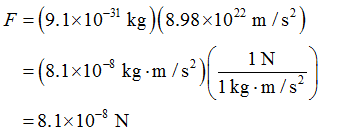Therefore, the force acting on the electron is of magnitude 8.1×10-8 N, and directed towards the center of the circular orbit.Question 2:-Wheel A of radius rA = 10.0 cm is coupled by a belt b to wheel C of radius rC = 25.0 cm, as shown in below figure. Wheel A increases its angular speed from rest at a uniform rate of 1.60 rad/s2. Determine the time for wheel C to reach a rotational speed of 100 rev/min, assuming the belt does not slip. (Hint: If the belt does not slip, the linear speeds at the rims of the two wheels must be equal.)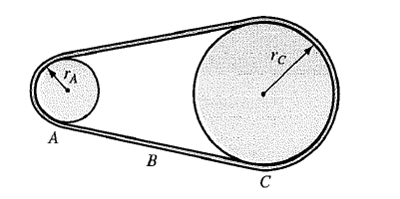Concept:Assume that desired angular speed of wheel C is ωC and the angular velocity of wheel A corresponding to angular speed of wheel C is ωA.

The belt rolls without slipping, therefore the tangential speed of the wheel must be equal, that is vTA = vTC

Here vTA is the tangential speed of wheel A and vTC is the tangential speed of wheel C.

The above equation can also be written as:

rAωA = rC ωC

ωA = (rC ωC) /rA

The time taken by wheel A to attain the angular speed of ωA is equal to the time taken by wheel C to attain the angular speed ωC. Therefore if the wheel has an angular acceleration say α, the time taken by the wheel A to move from rest to ωA is:

α = ωA/t

t = ωA/ α

Substitute ωA = (rC ωC) /rA in the equation t = ωA/ α,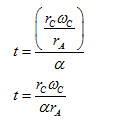Therefore the time taken by wheel C to attain the angular speed of ωC  is t =(rC ωC) /αrA.

Solution:

Substitute rA = 10.0 cm, rC = 25.0 cm, α = 1.60 rad/s2 and ωC = 100 rev/min in equation t   = (rC ωC) /αrA,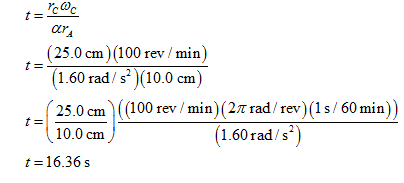Round off to three significant figures,

t = 16.4 s

Therefore the time taken by wheel C to attain the angular speed of 100 rev/min is 16.4 s.

Question 3:-Below figure shows a uniform block of mass, M and edge lengths a, b, and c. Calculate its rotational inertia about an axis through one corner and perpendicular to the large face of the block.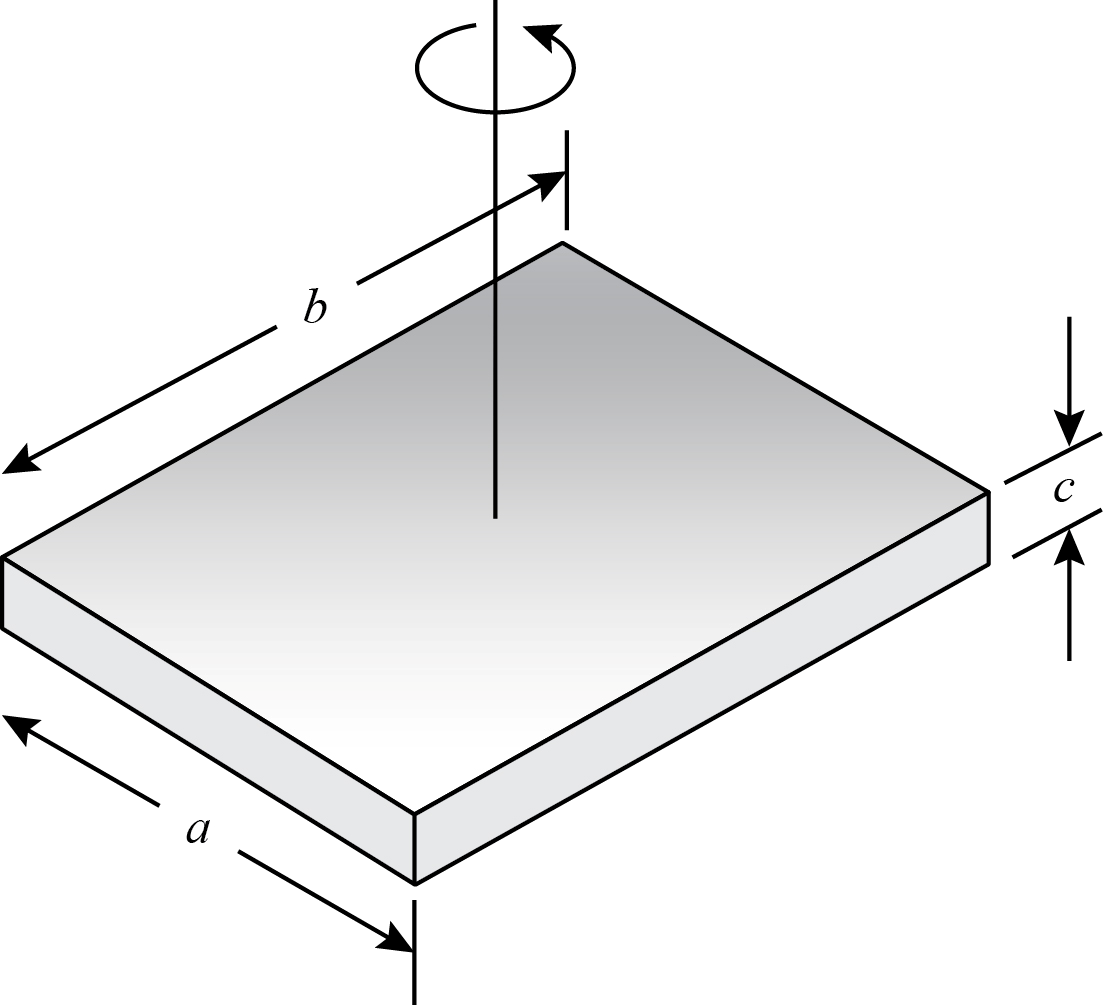Concept:

Use the rotational inertia of the uniform block about the axis passing through its center and normal to the plane, as shown in the diagram above:

The rotational inertia say I of the block about the axis shown in figure above is

1/12 [M (a2+b2)].

Therefore the rotational inertia (say I ') about the axis through one corner and perpendicular to the large face can be calculated using the parallel axis theorem as: I '= I +Mh2

Here h is the perpendicular distance between the both the axis (refer figure below)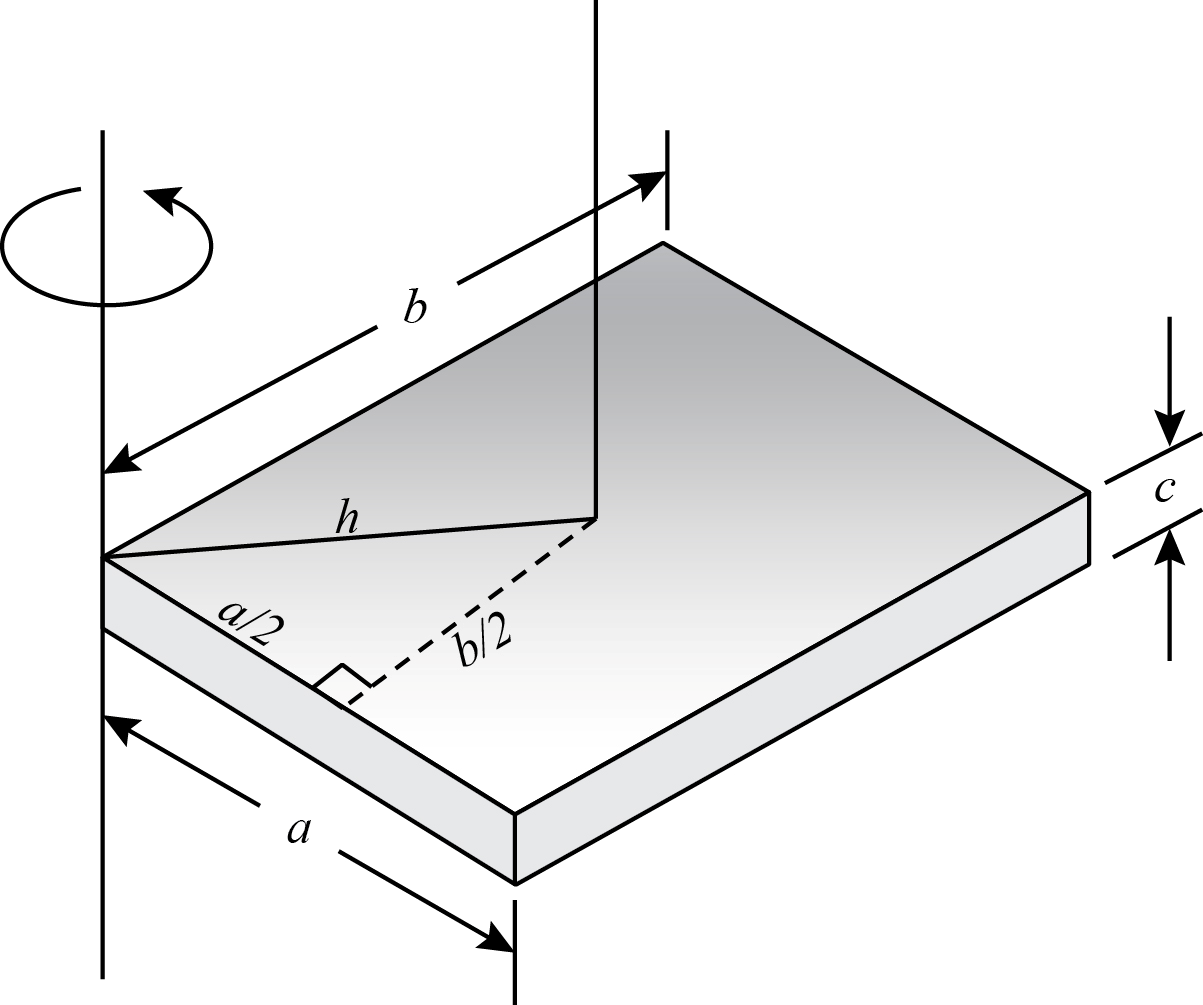Solution:

The distance h is given as: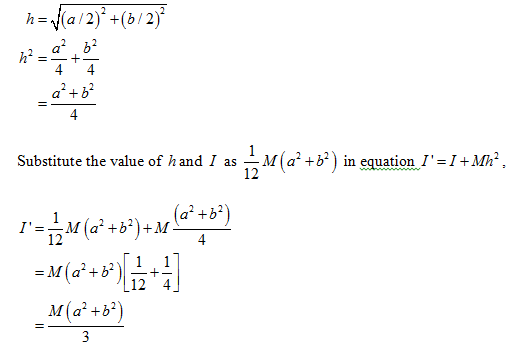Therefore the moment of inertia of the uniform block about the axis through one corner and perpendicular to large face of the block is M (a2 + b2)/3.

Question 4:- A top is spinning at 28.6 rev/s about an axis making an angle of 34.0º with the vertical. Its mass is 492 g and its rotational inertia is 5.12×10-4 kg.m2. The center of mass is 3.88 cm from the pivot point. The spin is clockwise as seen from above. Find the magnitude (in rev/s) and direction of the angular velocity of precession.

Concept:

The angular velocity of the precession of the spinning top is given as:

ω p = Mgr/L

Here L is the angular momentum of the spinning top, M is the mass of the spinning top, g is the acceleration due to gravity and r is the distance between the origin and the point at the top.

Also, the angular momentum of the spinning top can be written as L=Iω. Substitute the value of angular momentum in equation ω p = Mgr/L,

ω p = Mgr / Iω

Here ω is the angular velocity of the spinning top and I its rotational inertia.

Solution:

Substitute 492 g for M, 9.81 m/s2 for g, 3.88 cm for r, 5.12×10-4 kg.m2 for I and 28.6 rev/s for ω  in equation ω p = Mgr / Iω,Therefore, the angular velocity of the precession of the spinning top is 0.324 rev/s.

The figure below shows the spinning top and the direction of the angular velocity of precession.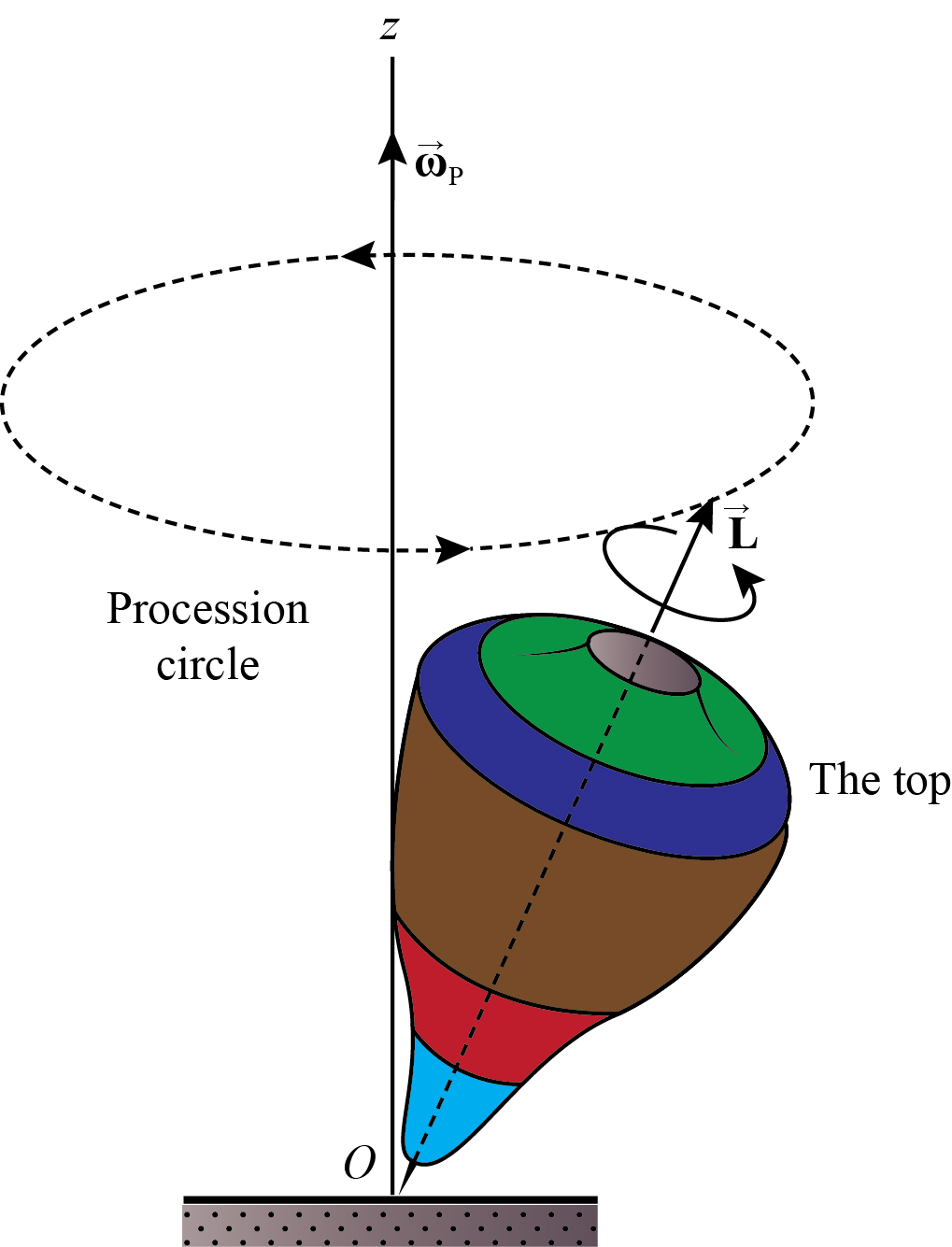It can be seen from the figure that the direction of ω p is perpendicular to the plane of cone.
```### Course Features

• 728 Video Lectures
• Revision Notes
• Previous Year Papers
• Mind Map
• Study Planner
• NCERT Solutions
• Discussion Forum
• Test paper with Video Solution